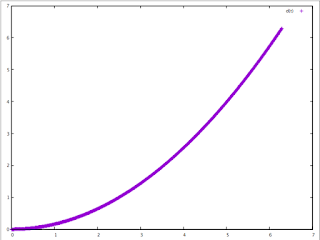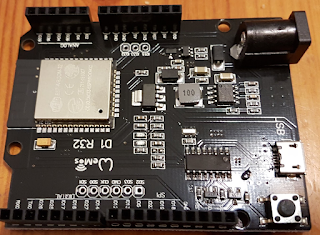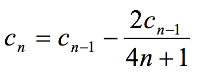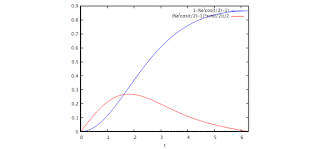## Posts

Showing posts from May, 2018

### One table to run them allIn previous entries, I have delved into the idea of using different shapes for the speed pattern for a motion using steppers and have presented how the timing can be based on a look-up table. Such a table would be pre-calculated and flashed with the firmware. But a single table provides a pattern for a given max speed, acceleration, number of steps and speed shape.

You can use that for timing the steps of the motor and it will accelerate properly as many steps as entries on your table. But it won't be practical if we want to change any of these parameters for other movements.
The idea I am not yet sure this works as expected, but first tests look ok, finally, as it took me a while to figure this out.
I have created a lookup table as the graph above, where each entry contains the delay needed for each step, for a movement of 1000 steps total, where speed will increase to 2Π while time goes till 2Π too. Maximum speed for each different motion pattern will be different though, but th…

### More experiments with motion curves for steppersThough I have been experimenting with Arduino, I realize that as soon as the computing requirements grow a bit the results quickly show the processor is actually slowing down my results. So now I am using a DOIT Espduino featuring an ESP32 processor that makes that sensitivity go away.

It was a bit of a challenge to get this board to work with CNCshield v3. The culprit was resistor R1 (enable pin) that would affect the booting capability of the ESP32. Once R1 was removed (brutally destroyed with a wire cutter in my case) the problem was gone and I could upload and run code with the Arduino IDE.

I created a simple program that uses some pre-calculated delays to perform the motion using different expressions for the acceleration. Four have been used and shown in the video below:

Uniform acceleration a=kSinusoidal acceleration a=1-cos(t)Exponential a=(e^(cos(t/2)-1)*sin(t/2))/2Exponential2 a=(e^(-(t-sin(t))/pi)*(1-cos(t)))/pi The expectation from a former entry was that adding the exponen…

### Stepper motor step signal timing calculationWhile we use stepper motors in many devices, the timing of the step signals is not always well understood. Most of us can see how a fixed speed is a bad idea unless the speed of motion is really slow, but it is fixed speed what is really to generate with simple code. You just can use the Arduino Blink example to send a fixed frequency of pulses to a stepper motor driver. If too slow, just reduce the delay value.

But for most real-life applications variable speed controls will perform better. These systems will speed up the motor to a given maximum speed and it will slow it down to stop at the desired step count. But understanding the math behind this process is sometimes not obvious.

Let us start with the simple case of the so-called trapezoidal speed motion profile. Here motion takes place in three phases: acceleration, constant speed (cruising) and deceleration. But the point is how can our code create such a behavior.

Stepper motor drivers usually accept two signals to control moto…

### A better stepper drive s-curve?One of the not so desirable features of stepper motors is the fact that output torque falls quickly with shaft speed. This is one of the reasons these motors cannot reach high speeds.

So I was thinking that when we use a s-curve to drive them, instead of choosing a symmetric one, where acceleration is is max in the middle of the acceleration section (as speed raises), I could try one where higher accelerations happen to lower speeds. However, in order to still keep the s-curve shape instead of a sawtooth, I would use the following expression: 1-e^(cos(t/2)-1)and it is shown in blue in the graph.

This will produce a s-curve shape, but modulated with the exponential behavior. Resulting signal will grow faster as the beginning (where more torque is available) and more slowly at the end (where faster speeds are reached and less torque is available).

A first test shows me that, for a bare motor, a faster move can be achieved, more reliably (no missed steps), using this pattern instead of …